Question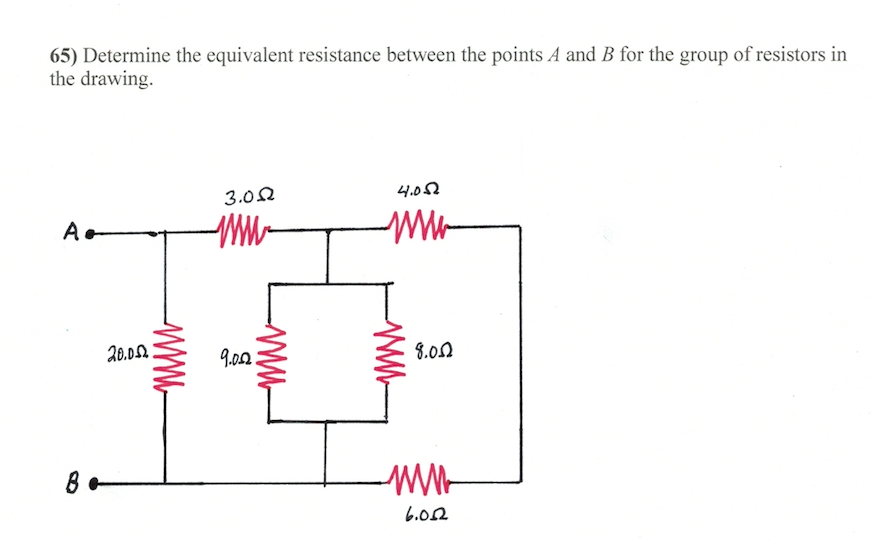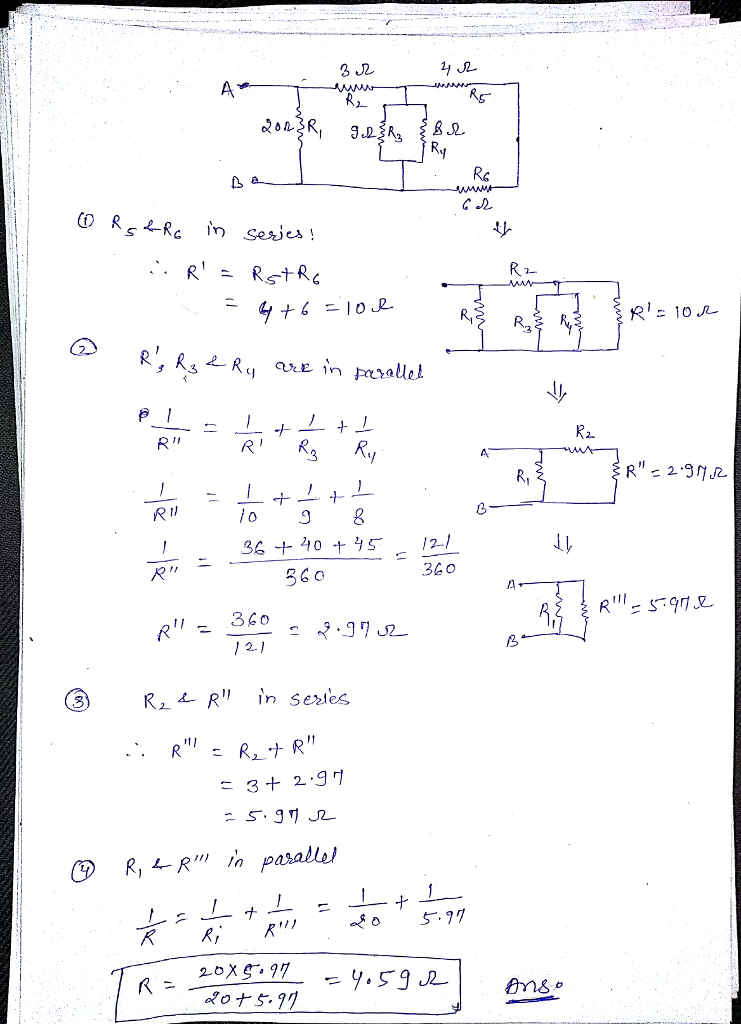#### Earn Coins

Coins can be redeemed for fabulous gifts.

Similar Homework Help Questions
• ### 9. Determine the equivalent resistance between the points A and B for the group of resistors...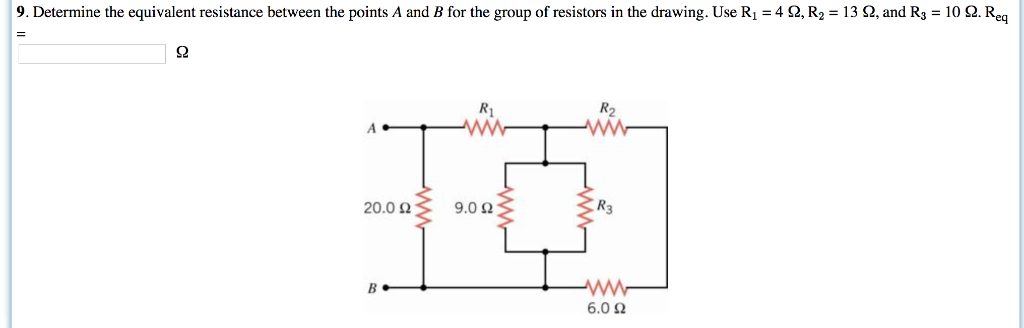9. Determine the equivalent resistance between the points A and B for the group of resistors in the drawing. Use R1-4 Ω, R2 = 13 Ω and R3 = 10 Ω Re Ri R2 20.0 Ω 9.0 Ω R3 6.0 Ω

• ### Find the equivalent resistance between points A and B in the drawing. 3.0 Ω 4.0 2...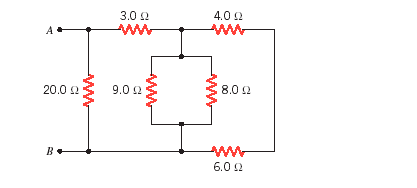Find the equivalent resistance between points A and B in the drawing. 3.0 Ω 4.0 2 8.0 Ω 20012 9.0 12 6.0 Ω

• ### What is the equivalent resistance of each group of resistors shown in the figure below? In...

What is the equivalent resistance of each group of resistors shown in the figure below? In the figure, R1 = 2.0 Ω, R2 = 3.0 Ω, R3 = 8.0 Ω, and R4 = 4.0 Ω. There is no figures present just Group A is in a series with R1 and R2 and R3. The secound Group is in a series with R4 and R4 and R4.

• ### Example 1: Four resistors are connected as shown (A) Find the equivalent resistance between points a...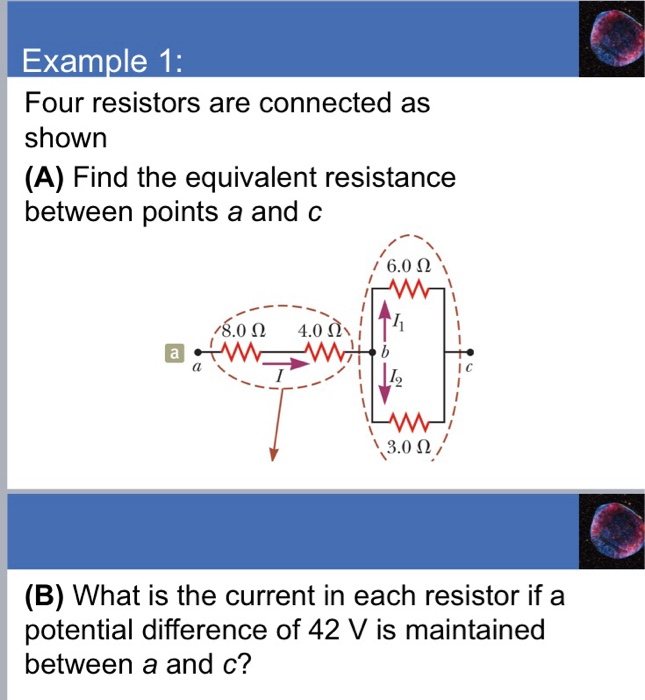Example 1: Four resistors are connected as shown (A) Find the equivalent resistance between points a and c /80 Ω 4.0(). IIT, I C 9 3,0 Ω (B) What is the current in each resistor if a potential difference of 42 V is maintained between a and c?

• ### Find the equivalent resistance between points A and B in the drawing. Find the equivalent resistance...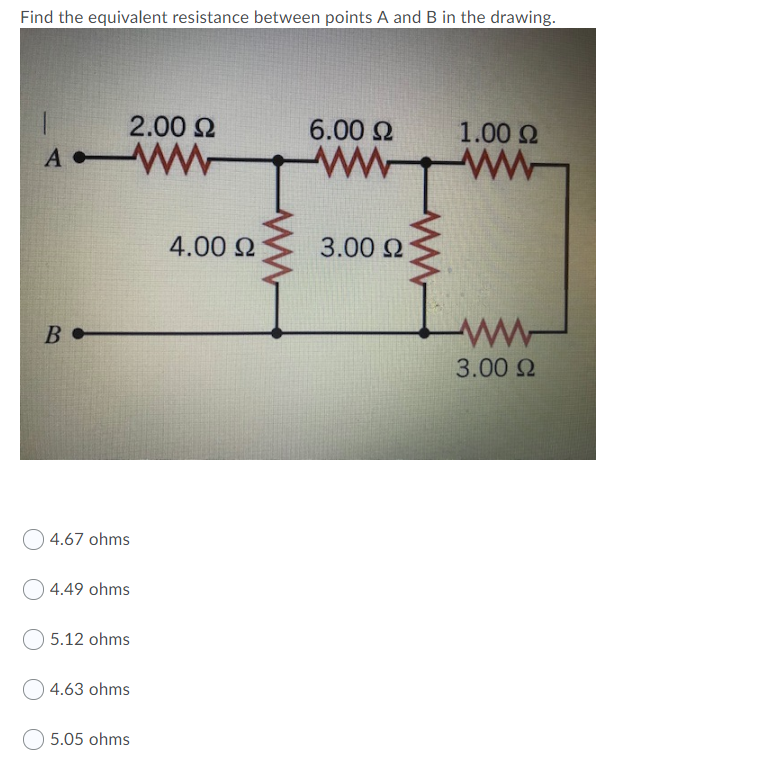Find the equivalent resistance between points A and B in the drawing. Find the equivalent resistance between points A and B in the drawing. 2.00 Ω 6.00 Ω 1.00 Ω A 4.00 Ω 3.00 Ω B - ΑΛΛ- 3.00 Ω 4.67 ohms Ο4.49 ohms 5.12 ohms 4.63 ohms 5.05 ohms

• ### What is the equivalent resistance between points a and b of the six resistors shown in the figure below?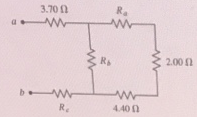What is the equivalent resistance between points a and b of the six resistors shown in the figure below? (Let Ra = 2.80 Ω, Rb = 5.00Ω, and Rc = 6.15 Ω.)

• ### Find the equivalent resistance between points A and B in the drawing. 3.0 4.0 A, a...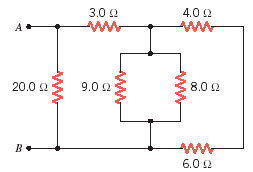Find the equivalent resistance between points A and B in the drawing. 3.0 4.0 A, a WW WW 20.0 9.0 2 8.0 6.0

• ### 4. Find the equivalent resistance between points A and B in the drawing (R1 = 4.0...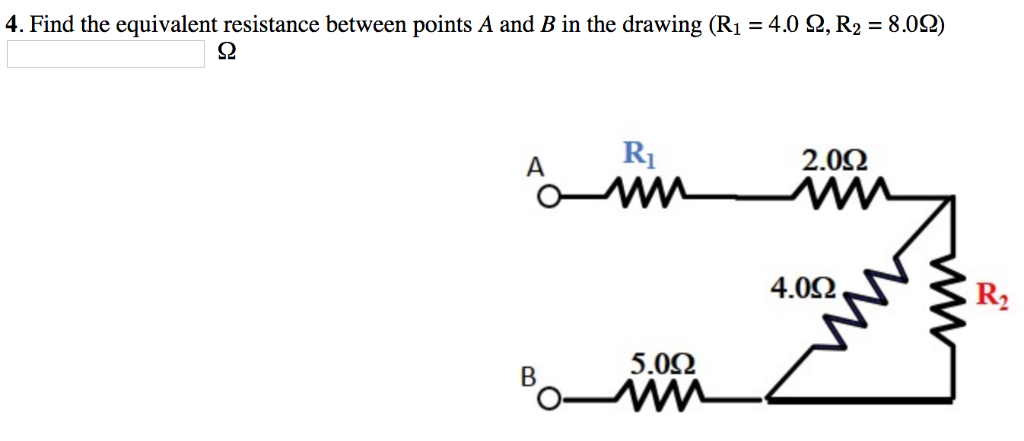4. Find the equivalent resistance between points A and B in the drawing (R1 = 4.0 Ω, R2 = 8.0Ω) S2 2.0Ω 4.0Ω R2 5.0Ω

• ### What is the equivalent resistance between points a and b of the six resistors shown in the figure below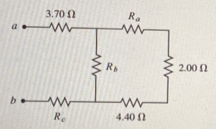What is the equivalent resistance between points a and b of the six resistors shown in the figure below? (Let Ra = 2.30Ω, Rb = 5.70Ω, and Rc = 6.30 Ω.)

• ### Determine the equivalent resistance of the "ladder" of equal 30 Ω resistors shown in figure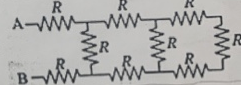Determine the equivalent resistance of the "ladder" of equal 30 Ω resistors shown in figure, in other words, what resistance would an ohmmeter read if connected between points A and B? b) (5 points) What is the power dissipated in the upper resistors on the left (the one connected to point A) if a 41 V battery is connected between points A and B?